# 2.1.1 Numerical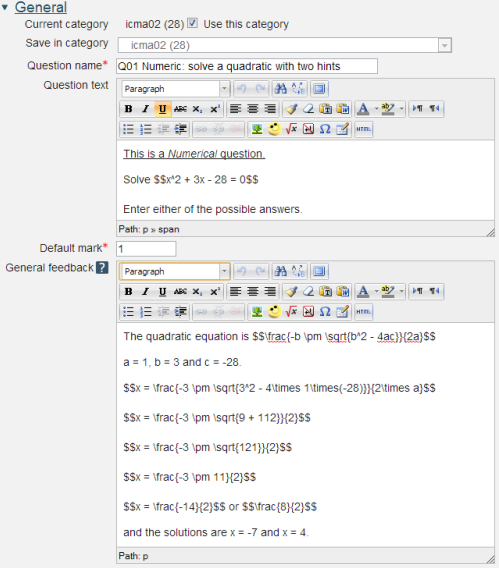2.3 The Question text, Default mark and General feedback. The text surrounded by two $symbols is LaTeX which will be interpreted by the maths filter and displayed in mathematical format. Question name: A descriptive name is sensible. This name will not be shown to students. Question text: You may use the full functionality of the editor to state the question. You may also include formatted mathematics using TeX or MathML. The text surrounded by two$ symbols in this example will be interpreted by the TeX filter and converted to nicely formatted mathematics. Please see the Including TeX and MathML section below.

## Placing the response box

The response box may be placed within the question rubric by including a sequence of underscores e.g. _____. At runtime the response box will replace the underscores and the length will be determined by the number of underscores. The minimum number of underscores required to trigger this action is 5. If no underscores are present the response box will be placed after the question and will be a full length box.

Default mark: Decide on how to score your questions and be consistent.

General feedback: We recommend that all questions should have this box completed with the correct answer and a fully worked explanation. The contents of this box will be shown to all students irrespective of whether their response was correct or incorrect. We do not recommend that authors rely on using the machine generated 'Right answer' (from the iCMA definition form) as this is:

• written by a computer
• frequently not the full right answer
• not necessarily in the correct format.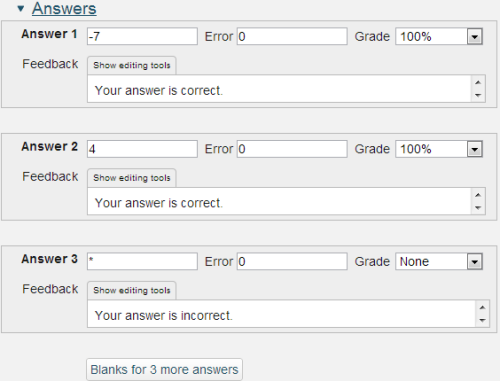Answer: an integer or decimal number. Use 'E' format for very large or very small values. '*' matches all other answers.

Error: Only rely on exact values for integers, for all other values specify a suitable range.

Feedback: Feedback that is specific to the answer.

Responses are matched in the order that they are entered into the form.

## A note on handling decimal numbers

If you are asking your user to enter an integer then it is OK to leave 'Error' empty. This means you are looking for the exact value.

But if you are expecting a non-integer value, or if any of your 'units' calculations will result in a non-integer answer, then you should be aware that the computer will treat the student's answer as a 'real', i.e. decimal, number. And because of the digital nature of computers and the finite number of 'bits' that are used to store 'reals' it is often the case that the real number cannot be held exactly; try writing 2/3 in decimal format where you only have 6 digits available and you'll see the problem. So if you are testing a 'real' value you should always use a small 'Accepted error'. In the example of 2/3, you may choose to look for a value of 0.665 and an 'Accepted error' of 0.0051, which will allow for both 0.66 or 0.67 and everything in-between such as 0.6667.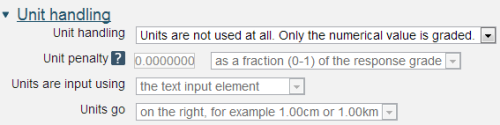2.5 Including units

The Numerical question type does not allow students to input powers and the Unit handling and Units sections provide ways around this. An alternative approach is provided in the Variable numeric with units question type.

Unit handling: a choice of three options:

• Units are not used at all. Only the numerical value is graded.
• Units are optional. If a unit is entered, it is used to convert the response to Unit 1 before grading
• The unit must be given and will be graded.

Unit penalty:

Units are input using: A choice of three options:

• the text input element
• a multiple choice selection

Units go: left or right.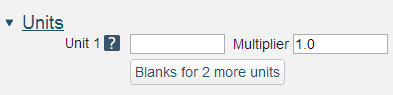2.6 Specifying units

The multiplier is the factor by which the correct numerical response will be multiplied.

The first unit (Unit 1) has a default multiplier of 1. Thus if the correct numerical response is 5500 and you set W as unit at Unit 1 which has 1 as default multiplier, the correct response is 5500 W.

If you add the unit kW with a multiplier of 0.001, this will add a correct response of 5.5 kW. This means that the answers 5500W or 5.5kW would be marked correct.

Note that the accepted error is also multiplied, so an allowed error of 100W would become an error of 0.1kW.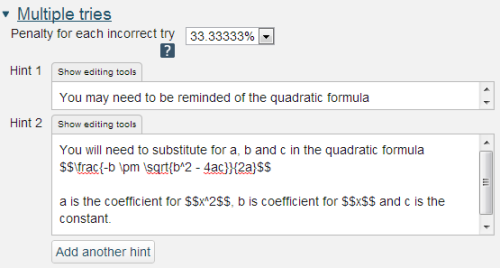2.7 Hints for use in Interactive with multiple tries behaviour

If you are configuring your question to run in 'Interactive with multiple tries' mode such that students can answer questions one by one you can also provide hints and second (and third) tries by entering appropriate feedback into the boxes provided in the 'Multiple tries' section of the question definition form.

To use this section of the question form please ensure that on the iCMA definition form you have chosen 'How questions behave' = 'Interactive with multiple tries'.

Penalty for each incorrect try: The available mark is reduced by the penalty for second and subsequent tries. In the example above a correct answer at the second try will score 0.6666667 of the available marks and a correct answer at the third try will score 0.3333334 of the available marks.

Hint: You can complete as many of these boxes as you wish. If you wish to give the student three tries at a question you will need to provide two hints. At runtime when the hints are exhausted the question will finish and the student will be given the general feedback and the question score will be calculated.

N.b. If you create a hint and then decide to delete it such that there is one less hint to the question you must ensure that all HTML formatting is also removed. To do this click on the 'HTML' icon in the editor toolbar which will display the HTML source. If any HTML formatting (in '< >') is present delete it and click on the HTML icon again to return to normal view.

2.1 Numeric response

2.1.2 Variable numeric